Next: MINIMUM K-EDGE CONNECTED SUBGRAPH Up: Cuts and Connectivity Previous: MINIMUM QUOTIENT CUT   Index

### MINIMUM K-VERTEX CONNECTED SUBGRAPH

• INSTANCE: Graph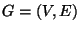. k is a constant,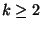.

• SOLUTION: A k-vertex connected spanning subgraph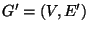for G, i.e. a spanning subgraph that cannot be disconnected by removing fewer than k vertices.

• MEASURE: The cardinality of the spanning subgraph, i.e.,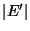.

• Good News: Approximable within 1+1/k  and .

• Comment: On directed graphs the problem is approximable within 1.61 for k=1 , and within 1+1/k for. Variation in which each edge has a nonnegative weight and the objective is to minimize the total weight of the spanning subgraph is approximable withinfor k=2  and within 2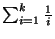for k>2 . If the weight function satisfies the triangle inequality, the problem is approximable within 3/2 for k=2  and within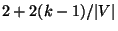for k>2 .

• Garey and Johnson: GT31

Viggo Kann
2000-03-20